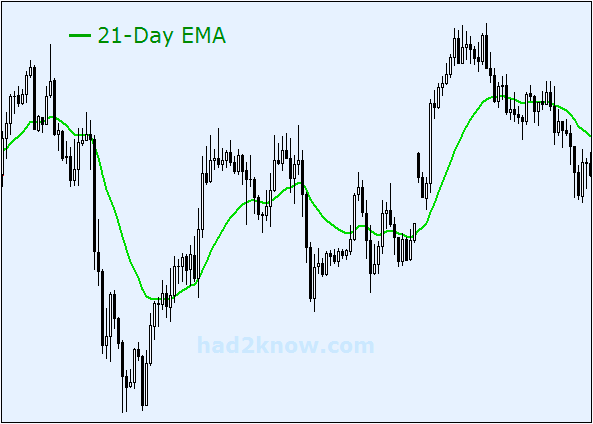# Exponential Moving Average Calculator

Given an ordered list of data points, you can construct the exponentially weighted moving average of all the points up to the current point. In an exponential moving average (EMA or EWMA for short), the weights decrease by a constant factor α as the terms get older. This kind of cumulative moving average is frequently used when charting stock prices. The recursive formula for EMA is

EMAtoday = α⋅xtoday + (1-α)EMAyesterday

where xtoday is today's current price point and α is some constant between 0 and 1. Often, α is a function of a certain number of days N. The most commonly used function is α = 2/(N+1). For instance, the 9-day EMA of a sequence has α = 0.2, while a 30-day EMA has α = 2/31 = 0.06452.

For values of α closer to 1, the EMA sequence can be initialized at EMA₁ = x₁. However, if α is very small, the earliest terms in the sequence may receive undue weight with such an initialization. To correct this issue in an N-day EMA, the first term of the EMA sequence is set to be the simple average of the first ⌈(N-1)/2⌉ terms, thus, the EMA starts on day number ⌈(N-1)/2⌉.

For instance, in a 9-day exponential moving average, EMA₄ = (x₁+x₂+x₃+x₄)/4. Then EMA₅ = 0.2x₅ + 0.8EMA₄ and EMA₆ = 0.2x₆ + 0.8EMA₅ etc.

 Enter comma-separated data here. DO NOT end list with a comma. Number of Days N:    2 3 4 5 6 7 8 9 10 11 12 13 14 15 20 21 25 28 30 35 40 50 60 100 Exponentially weighted moving average will be output here.

#### Using the Exponential Moving Average

Stock analysts often look at the EMA and SMA (simple moving average) of stock prices to note trends in the rise and fall of prices, and to help them predict future behavior. Like all moving averages, the highs and lows of the EMA graph will lag behind the highs and lows of the original unfiltered data. The higher the value of N, the smaller α will be and the smoother the graph will be.

Besides exponentially weighted cumulative moving averages, one can also calculate linearly weighted cumulative moving averages, in which the weights decrease linearly as the terms grow older. See the linear, quadratic, and cubic cumulative moving average article and calculator.